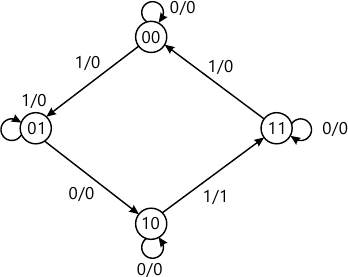Total marks: --
Total time: --
INSTRUCTIONS
(1) Assume appropriate data and state your reasons
(2) Marks are given to the right of every question
(3) Draw neat diagrams wherever necessary

1 (a) Simplify the following expression using Karnaugh-map. Implement the simplified expression using the gates as indicated.
f(a, b, c, d)=∑m (0,1,2,5,6,7,8,9,10,13,14,15) using only NAND gate
f(A, B,C,D)=Πm(0, 3, 4, 7, 8, 10, 12, 14)+∑d(2, 6) using only NOR gates.
12 M
1 (b) Design a logic circuit that has 4 inputs 4 inputs, the output will only be high, when the majority of the inputs are high use K map to simplify.
8 M

2 (a) Simplify using the Quine-McCluskey minimize technique. Implement the simplified expression using basic gates
V=f(a,b,c,d)=∑(2, 3, 4, 5, 13, 15)+∑d(8,9,10,11).
12 M
2 (b) Simplify the logic function given below using Variable Entered Mappings (VEM) techniques f(A, B, C, D)= ∑m(0, 1, 3, 5, 6, 11, 13)+∑d(4,7).
8 M

3 (a) With the aid of block diagram, clearly distinguish between a decoder and encoder.
4 M
3 (b) Design a combinational logic circuit that will convert a straight BCD digit to an excess-3 BCD digits
i) Construct the truth-table
ii) Simplify each output function using k map and write the reduced equations
iii) Draw the resulting logic diagram.
12 M
3 (c) Implement a full subtractor using a decoder and NAND gates.
4 M

4 (a) Implement the following Boolean function using 4:1 multiplexer F(A,B,C)=∑(1,3,5,6)
4 M
4 (b) Design a 2 bit comparator.
8 M
4 (c) What is a look ahead carry adder? Explain the circuit and operation of a 4 bit binary adder with look ahead carry.
8 M

5 (a) Differentiate sequential logic circuit and combinational logic circuit.
4 M
5 (b) Explain with timings diagram the working of a SR latch as a switch debouncer.
8 M
5 (c) Explain the working of a master-slave JK flip-flop with functional table and timings diagram.
8 M

6 (a) With the help of a diagram, explain the following with respect to shift register
i) Parallel in and serial out
ii) Ring counter and twisted rings counter.
8 M
6 (b) Explain the working of 4-bit asynchronous counter.
4 M
6 (c) Derive the characteristic equation of SR, JK, D and T flip-flops.
8 M

7 (a) With a suitable example, explain the mealy and Moore model of a sequential circuit.
10 M
7 (b) Design a synchronous counter using JK flip-flops to count the sequence 0,1,2,4,5,6,0,1,2 use static diagram and state table.
10 M

8 (a) Design a clocked sequential circuit that operates according to the state diagram shown. Implement the circuit using D-flip-flop.12 M
8 (b) With a suitable example and appropriate state diagram, explain how to recognize a particular sequence. EX 1011.
8 M

More question papers from Logic Design